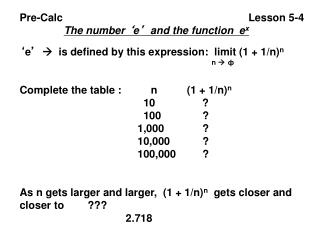DownloadDownload PresentationPre-Calc Lesson 5-4

Pre-Calc Lesson 5-4

Télécharger la présentationPre-Calc Lesson 5-4

- - - - - - - - - - - - - - - - - - - - - - - - - - - E N D - - - - - - - - - - - - - - - - - - - - - - - - - - -
Presentation Transcript

1. Pre-Calc Lesson 5-4 The number ‘e’ and the function ex ‘e’ is defined by this expression: limit (1 + 1/n)n n ф Complete the table : n (1 + 1/n)n 10 ? 100 ? 1,000 ? 10,000 ? 100,000 ? As n gets larger and larger, (1 + 1/n)n gets closer and closer to ??? 2.718

2. The Swiss mathematician Leonard Euler proved this concept which is why this ‘limit’ is called ‘e’ in his honor. The function ex is called the natural exponential function: Its graph looks like such: Compound Interest and the number ‘e’. ‘e’ is a very important number when computing interest. When computing interest on money at various Intervals  yearly, semi-annually, quarterly, monthly, even daily, we can use our basic formula for exponential

3. Growth---A(t) = A0(1 + r)t Now a few adjustments need to be made -- in this formula, ‘r’ stands for a ‘yearly interest rate. So if we want to compute interest on money at say 12% per year compounded semiannually—which means Two times a year—then we take ‘r’ 12% divide by two To get 6%, but then we take that to the 2nd power to get 1.062 = 1.1236 Confused??

4. Interest period % growth growth factor Amount each period during period . Annually 12% 1 + 0.12 1.121 = 1.12 1 . Semiannually 6% 1 + 0.12 1.062 = 1.1236 2 . Quarterly 3% 1 + 0.12 1.034 = 1.1255 4 . Monthly 1% 1 + 0.12 1.0112 = 1.1268 12 . Daily 12 % 1 + 0.12 (1 + 0.12)360 = 1.1275 (360 days) 360 360 360 . ‘k’ times 12 % 1 + 0.12 (1 + 0.12)k per year k k k . Through a series of mathematical manipulations, the expression (1 + 0.12)k can be written as [(1 + 1)n]0.12 k n Now we earlier stated that ‘e’ = (1 + 1)n n

5. So when we go to computing interest more often than • ‘daily’ referred to as ‘continuously’ we just use • A(t) = A0ert. Now since we usually are calculating interest • On money which is called ‘principle’ we use the ‘pert’ • Formula: A(t) = Pert. • Example: Which plan yields the most interest? • Plan A: A 7.5% annual rate compounded monthly • for 4 years • Plan B: A 7.2 % annual rate compounded daily for • 4 years • Plan C: A 7% annual rate compounded continuously • for 4 years

6. Plan A: (1 + 0.075)12(4) = (1.00625)48 = 1.3486 • 12 • Plan B: (1 + 0.072)360(4) = (1.0002)1440 = 1.3337 • 360 • Plan C: e0.07(4) = (2.7183)0.28 = 1.3231 • Plan A yields the most! • Use your calculator to evaluate the following: • e2 2. e3.2 3. e- 4 4. e 5. e1 • 6. Which is larger e or e

7. If money is invested at 8% compounded semi- • annually, then each year the investment is multiplied • by 1.042. What is the investment multiplied by if • the interest is compounded: • a) quarterly b) 12 times a year c) continuously • (1.02)4 • (1.0067)12 • e0.08 • A bank advertises that its 5% annual interest rate • compounded daily is equivalent to a 5.13 % effective • annual yield. What does this mean? • (1 + 0.05)360 = (1.000138889)360 = 1.051267… = 1.0513 • 360 • So 5 % compounded daily equates to approximately 5.13% • annually